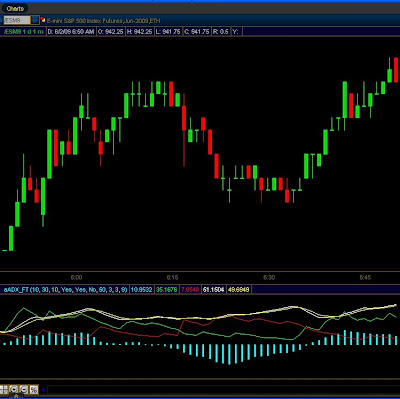## Tuesday, June 2, 2009

### ADX, DMI, MACD tri-indicator for one low price - FREEMany times a combination of indicators can shead more light on price movement. Try this combination and see how you like it. Change the input value invertNegMACD = Yes to see a more visual picture of MACD.

You can see ADX is colored White, and also ADX Average is colored Yellow. DMI(positive) is Green, DMI(Negative) is Red, and MACD is painted as a Histogram.declare lower;
input MACDfastLen = 10;
input MACDslowLen = 30;
input MACDLen = 10;
input showADX_DMI = { "No", default "Yes"};
input showMACD = { "No", default "Yes"};
input invertNegMACD = { "Yes", default "No"};
input MACDHeight = 50;
input MACDWidth = 3;
input DMI_Len = 9;

def fastAvg = Ema2(data = close, "smoothing factor" = 2 / (1 + MACDfastLen));
def slowAvg = Ema2(data = close, "smoothing factor" = 2 / (1 + MACDslowLen));
def Value = fastAvg - slowAvg;
def nextAvg = ExpAverage(data = Value, MACDLen);
def HistoBar = value - nextAvg;
def HiScale = HighestAll(HistoBar);
def LoScale = AbsValue(LowestAll(HistoBar));
def BarScale = if HiScale > LoScale then HiScale else LoScale;

plot macd_plot = if (showMACD, if( invertNegMACD, if ( HistoBar < 0,( -1 * HistoBar * MACDHeight / BarScale ),( HistoBar * MACDHeight / BarScale )),HistoBar * MACDHeight / BarScale), double.nan);

macd_plot.AssignValueColor(if invertNegMACD then if HistoBar >= 0 then Color.Cyan else Color.Magenta else Color.Cyan);
macd_plot.SetPaintingStrategy(PaintingStrategy.Histogram);
macd_plot.SetLineWeight(MACDWidth);

def hiDiff = high - high;
def loDiff = low - low;
def plusDM = if hiDiff > loDiff and hiDiff > 0 then hiDiff else 0;
def minusDM = if loDiff > hiDiff and loDiff > 0 then loDiff else 0;
def ATR = WildersAverage(TrueRange(high, close, low), DMI_Len);
plot "DI+" =
if showADX_DMI then 100 * WildersAverage(plusDM, DMI_Len) / ATR
else double.nan;
plot "DI-" =
if showADX_DMI then 100 * WildersAverage(minusDM, DMI_Len) / ATR
else double.nan;
def DX =
if ("DI+" + "DI-" > 0) then 100 * AbsValue("DI+" - "DI-") / ("DI+" + "DI-")
else 0;